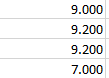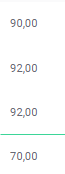# Datatable export to excel amount error``````    \$(document).ready(function () {
\$.fn.dataTable.moment(lang == "en" ? 'DD/MM/YYYY' : 'DD.MM.YYYY');
categories_table = \$('.datatable').DataTable({
order: [[1, 'desc']],
language: datatable_lang,
dom: 'Bfrtip',
buttons: [
{
extend: 'collection',
autoClose: 'true',
tag: 'span',
buttons: ['csv', 'print', 'excel']
}
]
});
});
``````

this way i call excel and others but data is wrong in excel. 90,00 in my table but 9,000 in excel. There is no problem in excel, I just need to add something in my code but I couldn't find it. My column is C. Sorry for my EnglishI assume you are using a decimal comma. There are two ways to resolve this.
a) you make your values not numeric by adding a % sign for example.
b) you are replacing the decimal commas with decimal points prior to passing them to Excel and format your columns to the right numeric format.

Excel doesn't understand decimal commas and periods as thousand separators. 90,00 is interpreted as a broken number because the comma can only be a thousand separator in America - which doesn't make sense if only two digits follow.

If you passed "1.900.000,76 EUR" Excel would not recognize this as a number but simply as text. Probably even if you drop the "EUR" from the string.

if your users don't need to do Excel calculations the easiest way to resolve the issue is solution a). Otherwise you would need to do something like this for example.

``````exportOptions: {
columns: excelColSelector,
modifier: { selected: null }, //make sure all records show up
format: {
body: function ( data, row, column, node ) {
if (typeof data === 'undefined') {
return;
}
if (data == null) {
return data;
}
if (\$.inArray(column, [...xlsTwoDecPlacesColsNumbers, ...xlsFourDecPlacesColsNumbers] ) >= 0) {
//                                if (column === 13) { //N !!
if (lang == 'de') { //this time we use the English formatting
//data contain only one comma we need to split there
var arr = data.split(',');
//subsequently replace all the periods with spaces
arr = arr.toString().replace( /[\.]/g, "" );
//join the pieces together with a period if not empty
if (arr > ''  || arr > '') {
data = arr + '.' + arr;
} else {
return '';
}
} else {
data = data.toString().replace( /[\,]/g, "" );
}
//result a number still as a string with decimal . and
//no thousand separators
//replace everything except numbers, decimal point and minus
data = data.toString().replace( /[^\d.-]/g, "" );
//percent must be adjusted to fraction to work ok
if (\$.inArray(column, xlsFourDecPlacesColsNumbers) >= 0) {
if (data !== '') {
data = data / 100;
}
}
return data;
}
//replace ampersands and other special chars
data = data.replace(/&gt;/g, '>')
.replace(/&lt;/g, '<')
.replace(/&amp;/g, '&')
.replace(/&quot;/g, '"')
.replace(/&#163;/g, '£')
.replace(/&#39;/g, '\'')
.replace(/&#10;/g, '\n');
//replace html tags with one space
data = data.replace(/<[^>]*>/g, ' ');
//replace multiple spaces and tabs etc with one space
data = data.replace(/\s\s+/g, ' ');
//limit length to 200 characters maximum
if ( data.length > 200 ) {
return data.substr(0, 197) + '...';
}
return data;
},
``````

I identify Excel column letters that contain numbers with two decimal places and numbers with four decimal places. The latter are percentages. Those numbers can come in German or American format depending on the user language. The code strips off the thousand separators and replaces an eventual decimal comma with a period.

So "1.900.000,76 EUR" becomes 1900000.76 when being sent to Excel.
That is what Excel understands. Depending on the client version of the user's Excel (German or American). The number is rendered to the user as 1.900.000,76 or 1,900,000.76 - if you get the cell styling right! Not an easy task.

That is done with "customize" like in here. As you can see this isn't trivial. So better stick to solution a) ...

``````customize: function( xlsx ) {
var sSh = xlsx.xl['styles.xml'];
var lastXfIndex = \$('cellXfs xf', sSh).length - 1;
var lastFontIndex = \$('fonts font', sSh).length - 1;
var f1 = //bold and underlined font
'<font>'+
'<sz val="11" />'+
'<name val="Calibri" />'+
'<b />'+'<u />'+
'</font>'

var i; var y;
//n1, n2 ... are number formats; s1, s2, ... are styles
var n1 = '<numFmt formatCode="##0.0000%" numFmtId="300"/>';
var s1 = '<xf numFmtId="300" fontId="0" fillId="0" borderId="0" applyFont="1" applyFill="1" applyBorder="1" xfId="0" applyNumberFormat="1"/>';
//define the style with the new font (number passed in as a variable)
var s2 = '<xf numFmtId="0" fontId="'+(lastFontIndex+1)+'" fillId="2" borderId="0" applyFont="1" applyFill="1" applyBorder="1" xfId="0" applyAlignment="1">'+
'<alignment horizontal="center"/></xf>';
var s3 = '<xf numFmtId="0" fontId="2" fillId="2" borderId="0" applyFont="1" applyFill="1" applyBorder="1" xfId="0" applyAlignment="1">'+
'<alignment horizontal="center" wrapText="1"/></xf>'
sSh.childNodes.childNodes.innerHTML += n1;  //new number format
sSh.childNodes.childNodes.innerHTML += f1; //new font
sSh.childNodes.childNodes.innerHTML += s1 + s2 + s3; //new styles

var fourDecPlaces    = lastXfIndex + 1;
var greyBoldCentered = lastXfIndex + 2;
var greyBoldWrapText = lastXfIndex + 3;

var sheet = xlsx.xl.worksheets['sheet1.xml'];
//create array of all columns (0 - N)
var cols = \$('col', sheet);

//two decimal places columns
for ( i=0; i < xlsTwoDecPlacesCols.length; i++ ) {
\$('row c[r^='+xlsTwoDecPlacesCols[i]+']', sheet).attr( 's', '64' );
}
//four decimal places columns
for ( i=0; i < xlsFourDecPlacesCols.length; i++ ) {
\$('row c[r^='+xlsFourDecPlacesCols[i]+']', sheet).attr( 's', fourDecPlaces );
}
//aktenzeichen und vertragsnummer as right aligned text
for ( i=0; i < xlsRightAlignedCols.length; i++ ) {
\$('row c[r^='+xlsRightAlignedCols[i]+']', sheet).attr( 's', '52' );
}

\$('row:eq(0) c', sheet).attr( 's', greyBoldCentered );  //grey background bold and centered, as added above
\$('row:eq(1) c', sheet).attr( 's', greyBoldWrapText );  //grey background bold, text wrapped
\$('row:last c', sheet).attr( 's', '2' );  //bold
},
``````
• It didnt help.I try but datatable is get error.